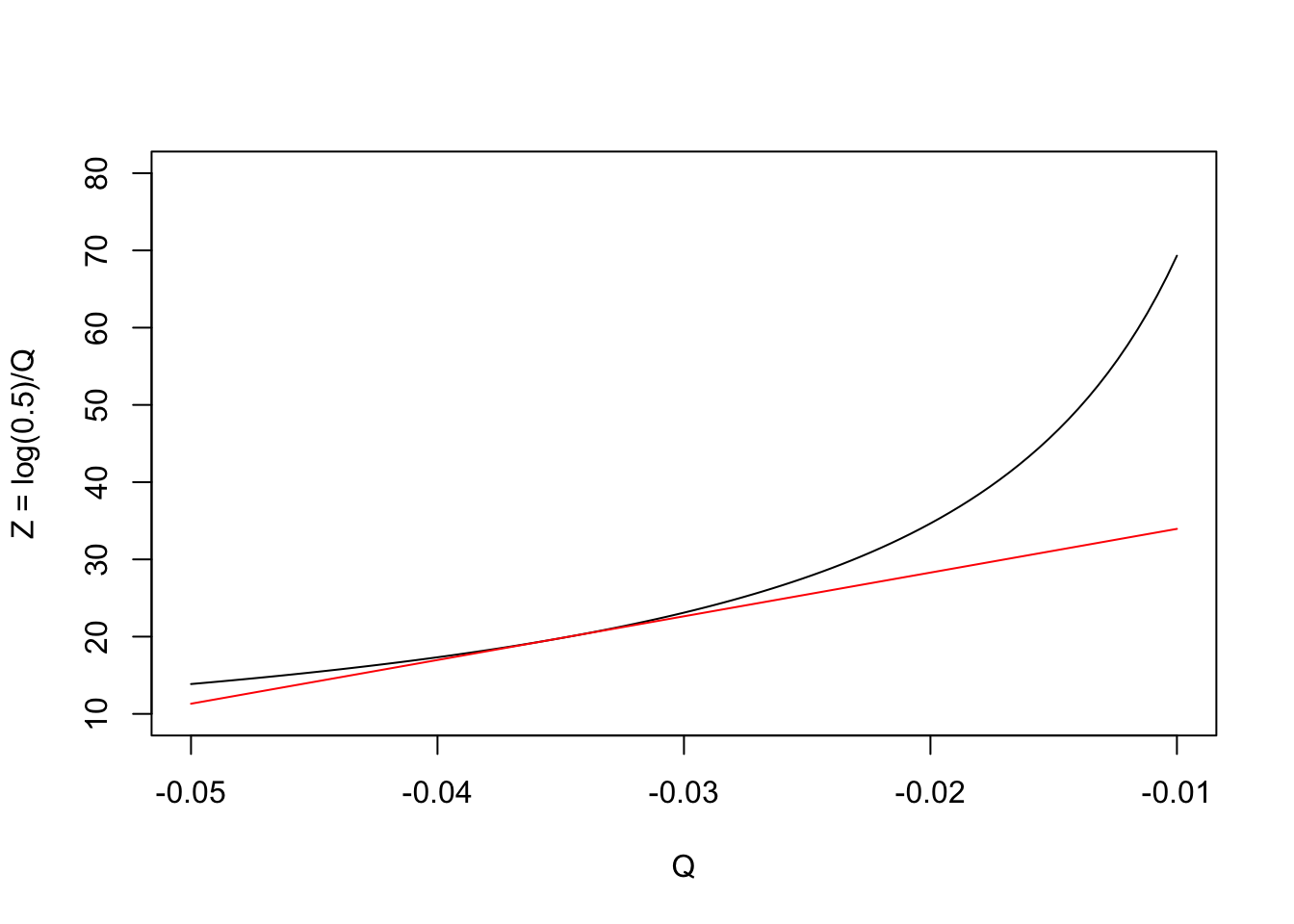# Articles by R on The broken bridge between biologists and statisticians

### How do we combine errors, in biology? The delta method

In a recent post I have shown that we can build linear combinations of model parameters (see here ). For example, if we have two parameter estimates, say Q and W, with standard errors respectively equal to $$\sigma_Q$$ and $$\sigma_W$$, we can build...### How do we combine errors, in biology? The delta method

In a recent post I have shown that we can build linear combinations of model parameters (see here ). For example, if we have two parameter estimates, say Q and W, with standard errors respectively equal to $$\sigma_Q$$ and $$\sigma_W$$, we can build a linear combination as follows: \[Z = ...### Dealing with correlation in designed field experiments: part II

With field experiments, studying the correlation between the observed traits may not be an easy task. Indeed, in these experiments, subjects are not independent, but they are grouped by treatment factors (e.g., genotypes or weed control methods) or by blocking factors (e.g., blocks, plots, main-plots). I have dealt ... [Read more...]

### Dealing with correlation in designed field experiments: part II

With field experiments, studying the correlation between the observed traits may not be an easy task. Indeed, in these experiments, subjects are not independent, but they are grouped by treatment factors (e.g., genotypes or weed control methods) or ... [Read more...]

### Dealing with correlation in designed field experiments: part I

April 29, 2019 |

Observations are grouped When we have recorded two traits in different subjects, we can be interested in describing their joint variability, by using the Pearson’s correlation coefficient. That’s ok, altough we have to respect some basic assumptions (e.g. linearity) that have been detailed elsewhere (see here). Problems ... [Read more...]

### Dealing with correlation in designed field experiments: part I

April 29, 2019 |

Observations are grouped When we have recorded two traits in different subjects, we can be interested in describing their joint variability, by using the Pearson’s correlation coefficient. That’s ok, altough we have to respect some basic assumption... [Read more...]

### How do we combine errors? The linear case

April 14, 2019 |

In our research work, we usually fit models to experimental data. Our aim is to estimate some biologically relevant parameters, together with their standard errors. Very often, these parameters are interesting in themselves, as they represent means, differences, rates or other important descriptors. In other cases, we use those estimates ... [Read more...]

### Some everyday data tasks: a few hints with R

March 26, 2019 |

We all work with data frames and it is important that we know how we can reshape them, as necessary to meet our needs. I think that there are, at least, four routine tasks that we need to be able to accomplish: subsetting sorting casting melting Obviously, there is a ... [Read more...]

### Drowning in a glass of water: variance-covariance and correlation matrices

February 18, 2019 |

One of the easiest tasks in R is to get correlations between each pair of variables in a dataset. As an example, let’s take the first four columns in the ‘mtcars’ dataset, that is available within R. Getting the variances-covariances and the corr...### Going back to the basics: the correlation coefficient

February 6, 2019 |

A measure of joint variability In statistics, dependence or association is any statistical relationship, whether causal or not, between two random variables or bivariate data. It is often measured by the Pearson correlation coefficient: \[\rho _{X,Y} =\textrm{corr} (X,Y) = \frac {\textrm{cov}(X,Y) }{ \sigma_X \sigma_Y } = \... [Read more...]

### My first experience with blogdown

November 14, 2018 |

This is my first day at work with blogdown. I must admit it is pretty overwhelming at the beginning … I thought that it might be useful to write down a few notes, to summarise my steps ahead, during the learning process. I do not work with blogdown everyday and I ... [Read more...]
1 2 3 4